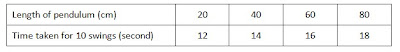## Monday, 16 August 2010

### Success Science

Paper 2 – Structured Question

Diagram below show the pendulum experiment.The table below show the result of the investigation.1. State the hypothesis tested in the experiment.
Tip: Hypothesis is the relationship between the manipulated variable [what is changed] and the responding variable [what is observed].

2. State the variables involved in this experiment.
i. Manipulated variable
ii. Responding variable
iii. Constant variable
Tip: The manipulated variable and responding variable can be obtained from the table. Constant variable means that if it is changed, the readings of the experiment will be change.

3. Estimate the time taken for the pendulum to make ten complete swings when the length of pendulum is 100cm.
Tip: Student must check the time interval.

4. State the relationship between the times taken for ten complete swings with its length.
Tip: Student have to check the pattern if it is increasing or decreasing.

5. Based on table above, sketch a graph of the pendulum length against the period of the pendulum swing.
Tip: If kind of graph is not stated, student should draw a line graph. Please use ruler to join the points. Mark the points clearly with an “x” symbol.